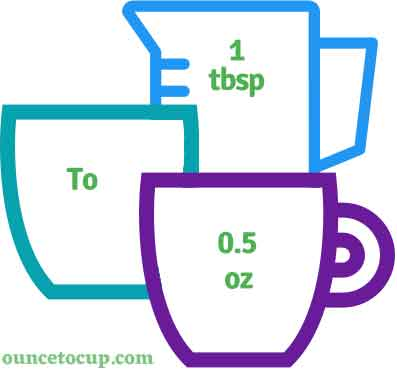# Tablespoon to Ounce (tbsp to oz conversion)

Are you cooking your favorite dish? The detailed chart in the recipe includes the calculation of the 1 tablespoon to ounce conversion.  Don't worry; use this calculator to determine how many 1 tablespoon equals ounces in a minute.  This 1 tbsp to oz converter gives an exact measurement for any recipe you prepare.

Tablespoon Value:

tbsp

Ounce Value:

oz

1 Tablespoon = 0.5 Ounce
(1 tbsp = 0.5 oz)

Try our auto 1 Tablespoon to Ounce Calculator (Without Convert Button), Just change the first field value and you got final value.## How many oz is a tbsp?

We know that the volume value of 1 tbsp is equal to 0.5 oz. If you want to convert 1 tbsp to an equal number of oz, just multiply the volume value by 0.5 or divide the value by 2. Hence, 1 tablespoon is equal to 0.5 oz.

The Answer is: 1 US Tablespoons = 0.5 US Ounce

1 tbsp = 0.5 oz

Many of them try to search or find an answer for what is 1 tablespoons in oz? So, we’ll start with 1 tbsp to oz conversion to know how big is 1 tbsp.

## How To Calculate 1 tbsp to oz?

To calculate 1 tablespoons to an equal number of ounce, simply follow the steps below.

Tablespoons to Ounces formula is:

FLuid Ounce = Tablespoon * 0.5

Assume that we are finding out how many oz were found in 1 fl tbsp of water, multiply by 0.5 to get the result.

Applying to Formula: oz = 1 tbsp * 0.5 = 0.5 oz.

## How To Convert tbsp to oz?

• To convert tablespoons to oz,
• Simply multiply the tablespoon value by 0.5.
• Applying to the formula, oz = 1 tablespoons * 0.5 [1x0.5] (or) simply divide them by 2.
• Hence, 1 tablespoons is equal to 0.5 oz.

## Some quick table references for tablespoon to ounce conversions:

Tablespoon [tbsp]Ounce [oz]
1 tbsp0.5 oz
2 tbsp1 oz
3 tbsp1.5 oz
4 tbsp2 oz
5 tbsp2.5 oz
6 tbsp3 oz
7 tbsp3.5 oz
8 tbsp4 oz
9 tbsp4.5 oz
10 tbsp5 oz
11 tbsp5.5 oz
12 tbsp6 oz
13 tbsp6.5 oz
14 tbsp7 oz
15 tbsp7.5 oz

## Reverse Calculation: How many tablespoons are in a oz?

• To convert 1 oz to tbsp,
• Simply mutiply the 1 oz by 2.
• Then, applying the formula, tbsp = 1 oz * 2 [1x2 = 2].
• Hence, 1 oz is equal to 2 tbsp.

### Related Converter:

Formula: tablespoon to oz

oz = tablespoon * 0.5

Applying to Formula,

oz = 1*0.5 = 0.5

1 tbsp = 0.5 oz